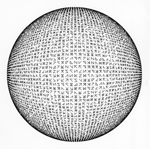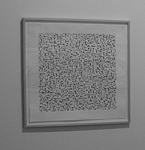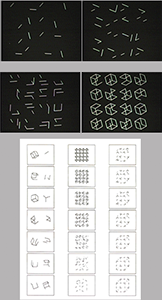Below are some of my early drawings - square and cube drawings formed from linear transformations, squares with its 4 lines (and 2 diagonals) used as an alphabet, triangles with its 3 lines used as an alphabet, algorithms which add and subtract lines to form structures - 1970-1973 which are directly related to my drawings from Cubic Limit I & II, Cubes with its 12 lines used as an Alphabet, 1973 - 1976 Click on any image to obtain more information about the drawings (larger images, algorithms, first published)Computer algorithmic drawings with Square generated by a Linear Transformation, 1970 Computer algorithmic drawings with Cubes generated from Linear Transformations, 1971Computer algorithmic plotter drawings generated from the 4 edges of a Square (and its 2 diagonals) as an alphabet, 1972Computer algorithmic plotter drawings generated from the 3 edges of a Triangle as an alphabet, 1973Early algorithms which add and subtract lines, 1970EarlyComputer algorithmic plotter drawings generated from the 12 edges of a Cube as an alphabet, 1973 Parts of computer generated films "cubic limit" and "complimentary cubes" with 12 lines of Cube as an alphabet made in 1973 All Alphabets, 1970 - 1973